SSAT Elementary Level Math : How to find the decimal equivalent of a fraction

Example Questions

What is the decimal equivalent of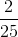?

.084

.40

.044

.04

.08

.08

Explanation:

Approach 1: Divide the numerator, 2, by the denominator, 25, to get a decimal equivalent of .08.

Approach 2: Manipulate the fraction so that it has a denominator of 100, and then determine the decimal answer based on the numerator.

To get a denominator of 100, multiply both the numerator and denominator by 4: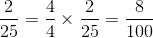The numerator, 8, can also be written as 8.0. Now move the decimal two places to the right to get the answer: .08

Example Question #82 : Ssat Elementary Level Quantitative (Math)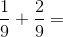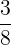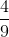Explanation:

Add fractions with the same denominator by adding their numerators and keeping the denominator the same: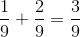The resulting fraction can be reduced because both the numerator and denominator are multiples of 3: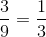Example Question #83 : Ssat Elementary Level Quantitative (Math)

What isequal to in decimal form?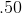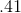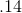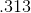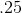Explanation:

To find the decimal equivalent of a fraction, simply divide the top number by the bottom number. In this problem,divided byequalsExample Question #51 : How To Find The Decimal Equivalent Of A Fraction

What is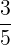equal to in decimal form?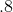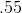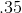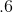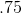Explanation:

To find the decimal equivalent of a fraction, simply divide the top number by the bottom number. In this problem,divided byequals.

Example Question #8 : Other Fraction Manipulations

Which fraction is less than?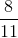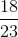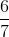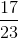Explanation:

The best way to answer this question is to find the smallest fraction among the answer choices.

Comparing fractions can be done easily when they are converted to decimals.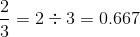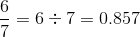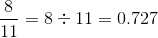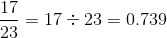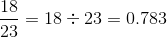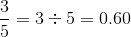Of these options, only, or 0.60, is less than.

Example Question #51 : How To Find The Decimal Equivalent Of A Fraction

What is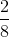equal to in decimal form?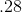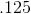Explanation:

To find the decimal equivalent of a fraction, simply divide the top number by the bottom number. In this problem,divided byequalsExample Question #51 : How To Find The Decimal Equivalent Of A Fraction

What is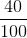equal to in decimal form?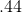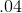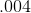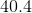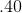Explanation:

To find the decimal equivalent of a fraction, simply divide the top number by the bottom number. In this problem,divided byequalsExample Question #52 : How To Find The Decimal Equivalent Of A Fraction

What is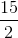equal to in decimal form?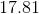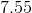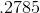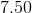Explanation:

To find the decimal equivalent of a fraction, simply divide the top number by the bottom number. In this problem,divided byequalsEven though the numerator (top number) is larger than the denominator (bottom number), the method for obtaining the decimal equivalent is the same as when the denominator is larger.

Example Question #53 : How To Find The Decimal Equivalent Of A Fraction

What is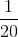equal to in decimal form?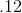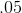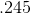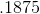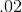Explanation:

To find the decimal equivalent of a fraction, simply divide the top number by the bottom number. In this problem,divided byequalsExample Question #55 : How To Find The Decimal Equivalent Of A Fraction

What isequal to in decimal form?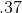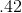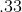To find the decimal equivalent of a fraction, simply divide the top number by the bottom number. In this problem,divided byequals